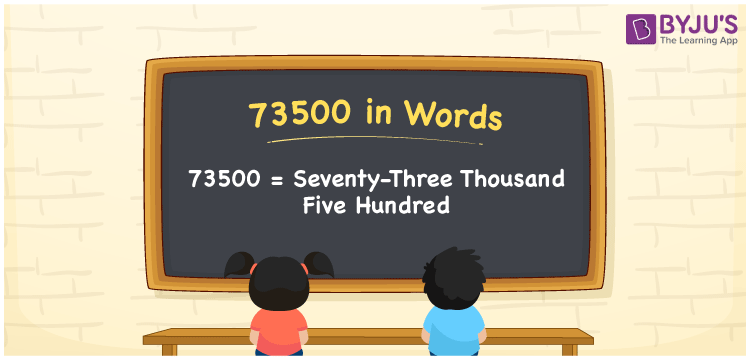# 73500 in Words

73500 in words is written as Seventy-three thousand five hundred. In both the International System of Numerals and the Indian System of Numerals, 73500 is written as Seventy-three thousand five hundred. The number 73500 is a Cardinal Number as it represents some quantity. For example, “that laptop costs 73500 rupees”.

 73500 in Words Seventy-three thousand five hundred Seventy-three thousand five hundred in Number 73500

## 73500 in English Words

73500 in English words is read as “Seventy-three thousand five hundred.”## How to Write 73500 in Words?

To write 73500 in words, we shall use the place value chart. In the place value chart, put 7 in the ten thousands, 3 in the thousands, 5 in the hundreds and put 0 in the rest. Let us make a place value chart to write the number 73500 in words.

 Ten Thousands Thousands Hundreds Tens Ones 7 3 5 0 0

Thus, we can write the expanded form as

7 × Ten Thousand + 3 × Thousand + 5 × Hundred + 0 × Ten + 0 × One

= 7 × 10000 + 3 × 1000 + 5 × 100 + 0 × 10 + 0 × 1

= 70000 + 3000 + 500 + 0 + 0

= 73500

= Seventy-three thousand five hundred.

73500 is a natural number, the successor of 73499 and the predecessor of 73501.

73500 in words – Seventy-three thousand five hundred

• Is 73500 an odd number? – No
• Is 73500 an even number? – Yes
• Is 73500 a perfect square number? – No
• Is 73500 a perfect cube number? – No
• Is 73500 a prime number? – No
• Is 73500 a composite number? – Yes

## Frequently Asked Questions on 73500 in Words

Q1

### How to write 73500 in words?

73500 in words is written as Seventy-three thousand five hundred.
Q2

### How to write 73500 in the International and Indian System of Numerals?

In both, the system of numerals, 73500 in words, is written as Seventy-three thousand five hundred.
Q3

### How to write 73500 in a place value chart?

In the place value chart, write 7 in the ten thousands, 3 in the thousands, 5 in the hundreds and 0 in the tens and the ones, respectively.